Question

# 1.A cantilever AD is subjected to a pure moment wa at B and uniformly distributed load of intensity w along AB and BC and as shown in Figure 1. The beam has constant EI. Ignore the weight of the bean...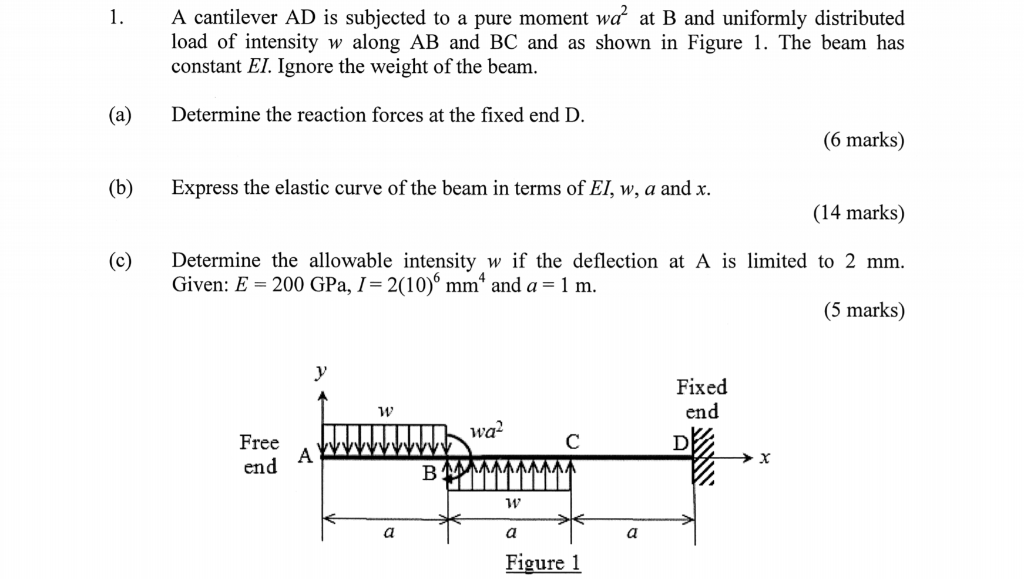1.A cantilever AD is subjected to a pure moment wa at B and uniformly distributed load of intensity w along AB and BC and as shown in Figure 1. The beam has constant EI. Ignore the weight of the beanm (a)Determine the reaction forces at the fixed end D. (6 marks) (b) Express the elastic curve of the beam in terms of EI, w, a and x. (14 marks) (c)Determine the allowable intensity w if the deflection at A is limited to 2 mm (5 marks) Given: E 200 GPa, 1 2(10)6 mm* and a 1 m Fixed en wa Free en Figure 1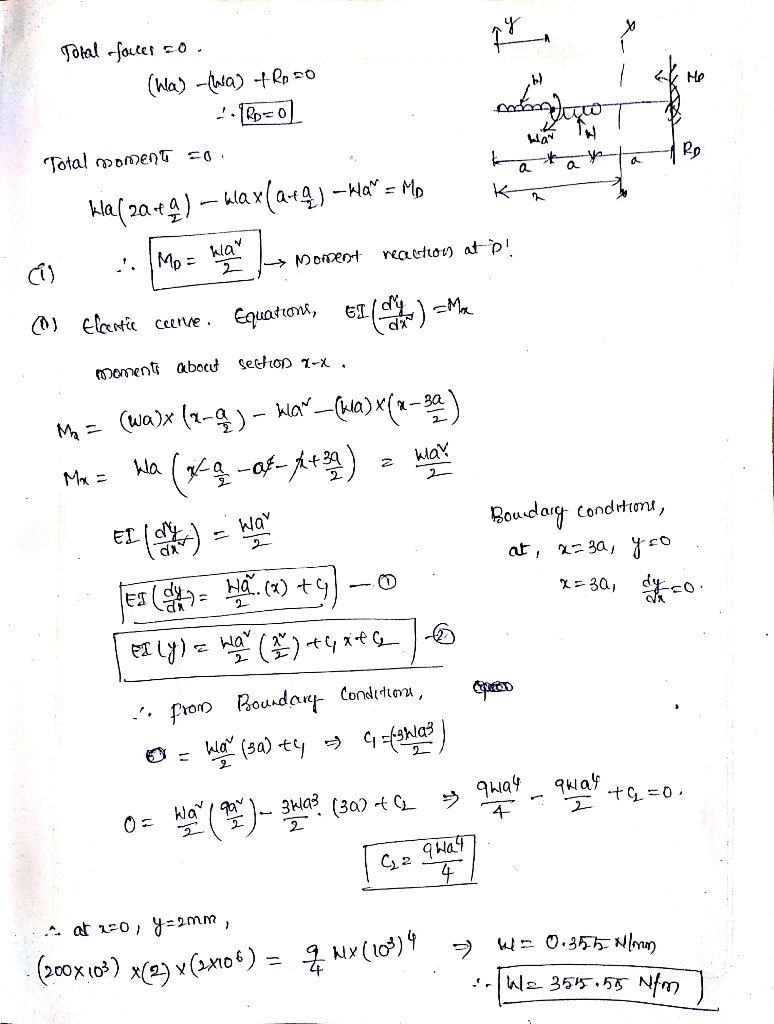#### Earn Coins

Coins can be redeemed for fabulous gifts.

Similar Homework Help Questions
• ### Q2 The 10 m long simply supported beam is subjected to a uniformly distributed load w...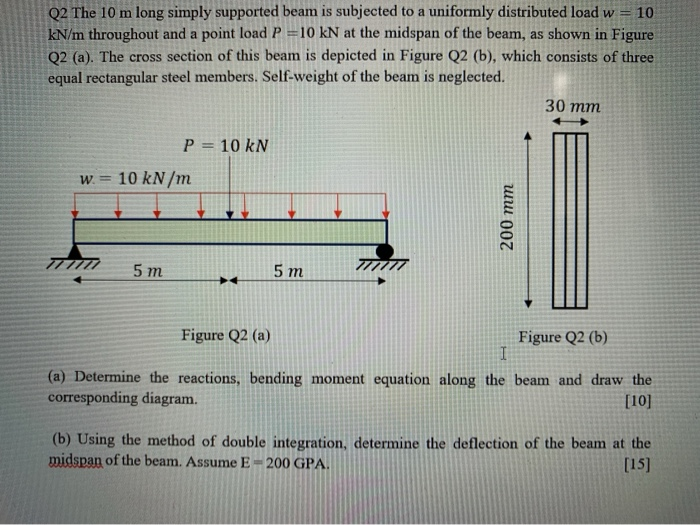Q2 The 10 m long simply supported beam is subjected to a uniformly distributed load w = 10 kN/m throughout and a point load P =10 kN at the midspan of the beam, as shown in Figure Q2 (a). The cross section of this beam is depicted in Figure Q2 (b), which consists of three equal rectangular steel members. Self-weight of the beam is neglected. 30 mm P= 10 KN W = 10 kN/m 200 mm 5 m 5 m...

• ### Q2 The 10 m long simply supported beam is subjected to a uniformly distributed load w...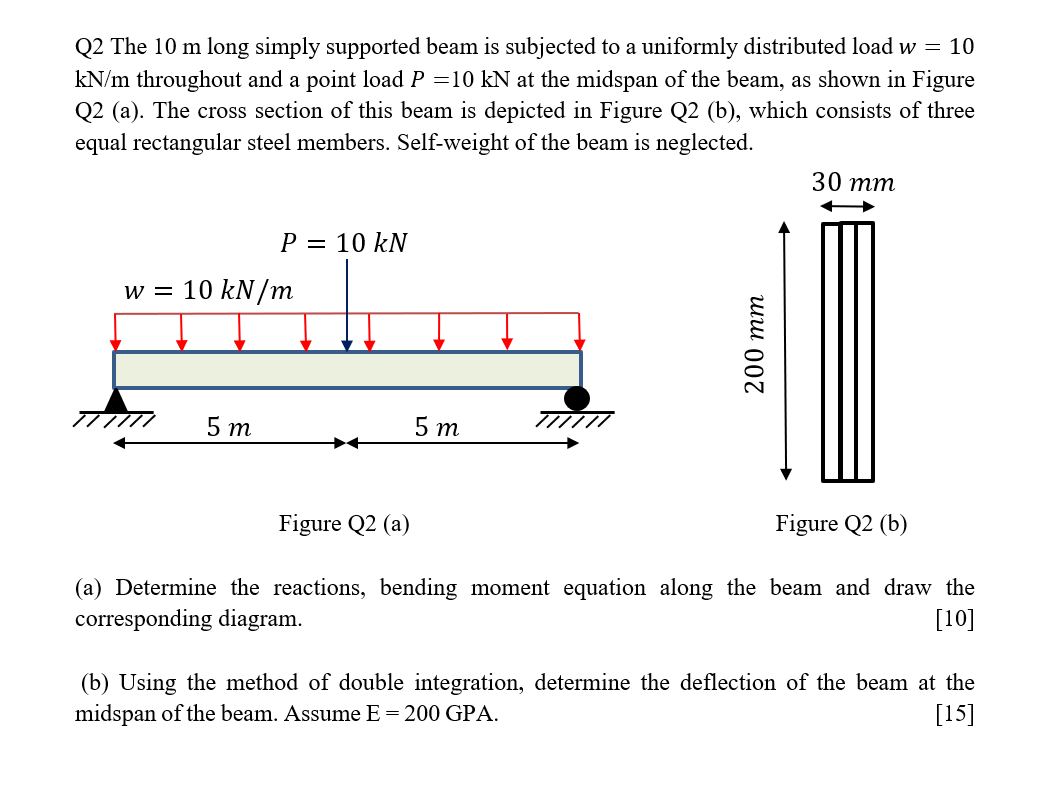Q2 The 10 m long simply supported beam is subjected to a uniformly distributed load w = 10 kN/m throughout and a point load P =10 kN at the midspan of the beam, as shown in Figure Q2 (a). The cross section of this beam is depicted in Figure Q2 (b), which consists of three equal rectangular steel members. Self-weight of the beam is neglected. 30 mm P = 10 kN W = 10 kN/m 200 mm 5 m 5...

• ### Q2 The 10 m long simply supported beam is subjected to a uniformly distributed load w...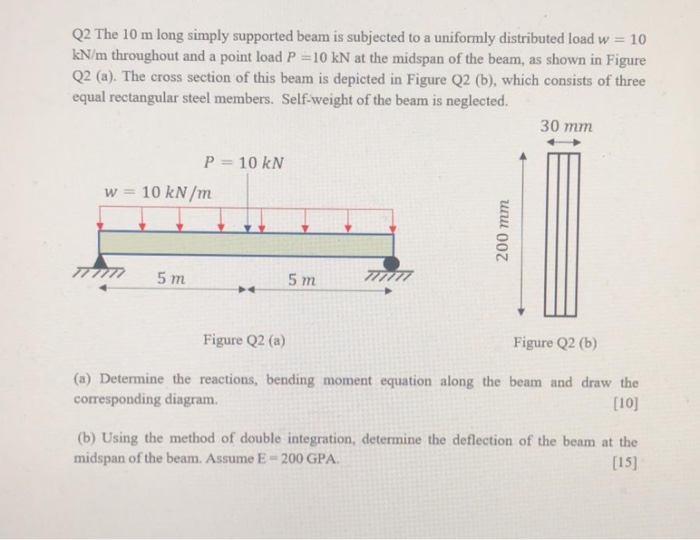Q2 The 10 m long simply supported beam is subjected to a uniformly distributed load w = 10 kN/m throughout and a point load P =10 kN at the midspan of the beam, as shown in Figure Q2 (a). The cross section of this beam is depicted in Figure Q2 (b), which consists of three equal rectangular steel members. Self-weight of the beam is neglected. 30 mm P = 10 kN w = 10 kN/m 200 mm 5 m 5...

• ### Q2. A simply supported beam AB (Figure 2) supports a uniformly distributed load of q =...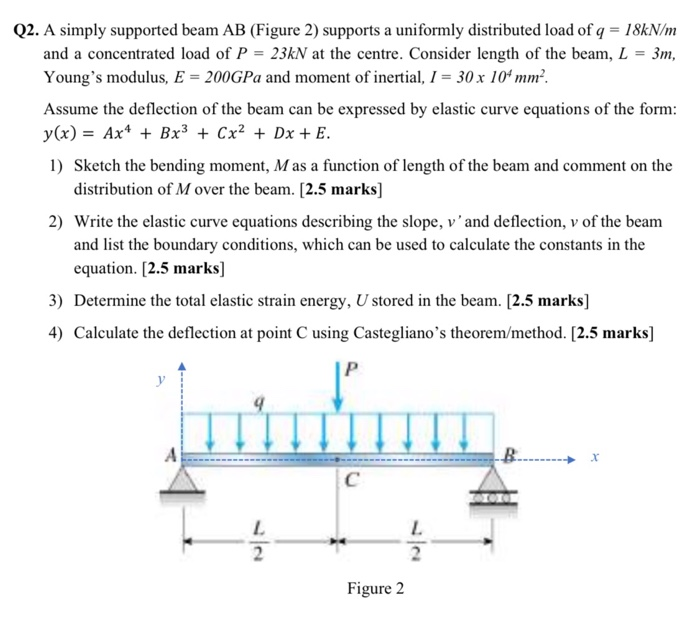Q2. A simply supported beam AB (Figure 2) supports a uniformly distributed load of q = 18kN/m and a concentrated load of P = 23kN at the centre. Consider length of the beam, L = 3m, Young's modulus, E = 200GPa and moment of inertial, I = 30 x 10 mm-. Assume the deflection of the beam can be expressed by elastic curve equations of the form: y(x) = Ax4 + Bx3 + Cx2 + Dx + E. 1) Sketch...

• ### Q.2) A boxed beam shown below supports a uniformly distributed load w 180 N/m. Two parts of the b...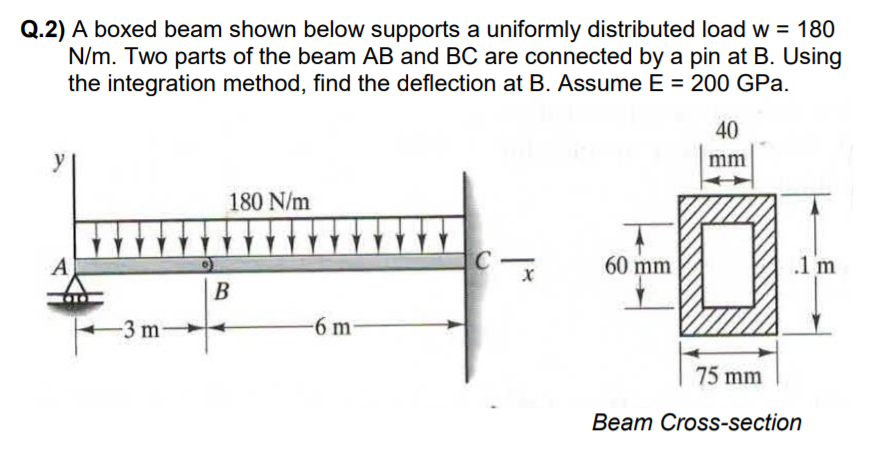Q.2) A boxed beam shown below supports a uniformly distributed load w 180 N/m. Two parts of the beam AB and BC are connected by a pin at B. Using the integration method, find the deflection at B. Assume E = 200 GPa. 40 180 N/m 60 mm 1 m 75 mm Beam Cross-section Q.2) A boxed beam shown below supports a uniformly distributed load w 180 N/m. Two parts of the beam AB and BC are connected by a...

• ### Q5. The cantilever beam, AC, is subjected to the load case shown in Figure 5. For the loading shown, do the following: [10 Marks] a) Calculate the magnitude and direction of the reactions at A b) Usi...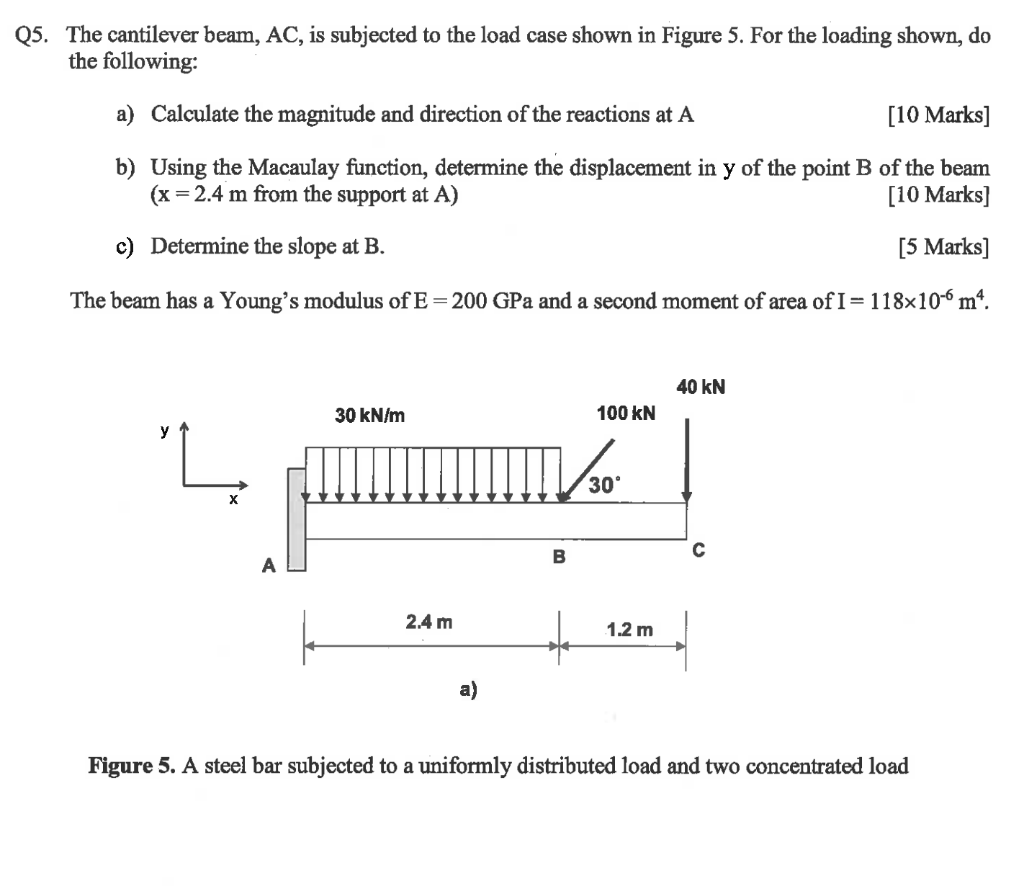Q5. The cantilever beam, AC, is subjected to the load case shown in Figure 5. For the loading shown, do the following: [10 Marks] a) Calculate the magnitude and direction of the reactions at A b) Using the Macaulay function, determine the displacement in y of the point B of the beam (x 2.4 m from the support at A) [10 Marks] c) Determine the slope at B. [5 Marks] The beam has a Young's modulus of E-200 GPa and...

• ### strength of material Final Exam Secoud Semester 201 Q1: A as shown in fig (1), cantilever beam AB carries a uniformly distributed load W per lengths (100 KNm) E=200 Gpa Determine the compressive a...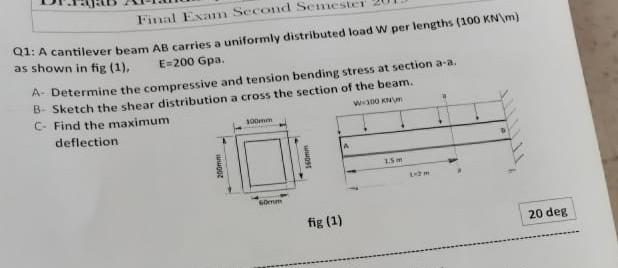strength of material Final Exam Secoud Semester 201 Q1: A as shown in fig (1), cantilever beam AB carries a uniformly distributed load W per lengths (100 KNm) E=200 Gpa Determine the compressive and tension bending stress at section a-a. B- Sketch the shear distribution a cross the section of the beam. C- Find the maximum A- 00mm deflection 1.sm fig (1) 20 deg Final Exam Secoud Semester 201 Q1: A as shown in fig (1), cantilever beam AB carries...

• ### 1. (19 pts) The cantilever beam is subjected to a distributed load w (unit N/m) as...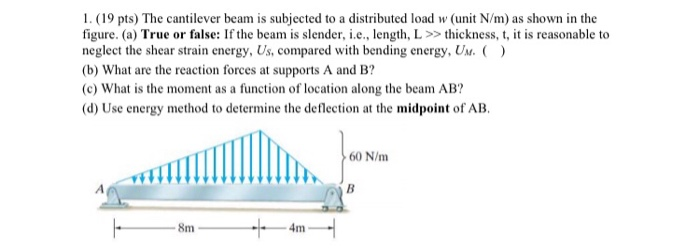1. (19 pts) The cantilever beam is subjected to a distributed load w (unit N/m) as shown in the figure. (a) True or false: If the beam is slender, i.e., length, L >> thickness, t, it is reasonable to neglect the shear strain energy, Us, compared with bending energy, UN. ) (b) What are the reaction forces at supports A and B? (c) What is the moment as a function of location along the beam AB? (d) Use energy method...

• ### The overhang beam is subjected to the uniform distributed load having an intensity of w = 46 kN/m (Figure 1)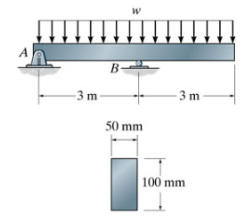The overhang beam is subjected to the uniform distributed load having an intensity of w = 46 kN/m (Figure 1) Part A Determine the maximum shear stress developed in the beam.

• ### 2.- The beam shown in the figure, has a roller support at A, a fixed support at C and an internal hinge at B. The lengths of segments AB and BC are and b respectively. A uniformly distributed load, q...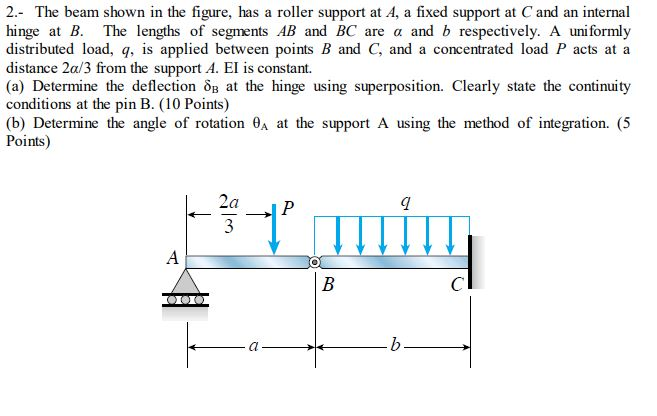2.- The beam shown in the figure, has a roller support at A, a fixed support at C and an internal hinge at B. The lengths of segments AB and BC are and b respectively. A uniformly distributed load, q, is applied between points B and C, and a concentrated load P acts at a distance 2a/3 from the support A. El is constant. (a) Determine the deflection o at the hinge using superposition. Clearly state the continuity conditions at...